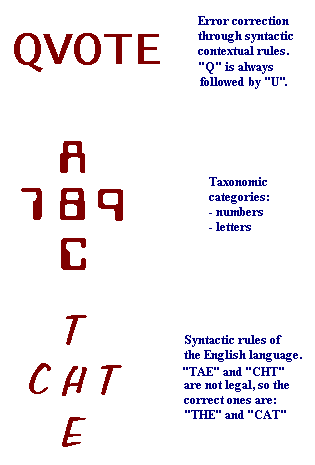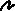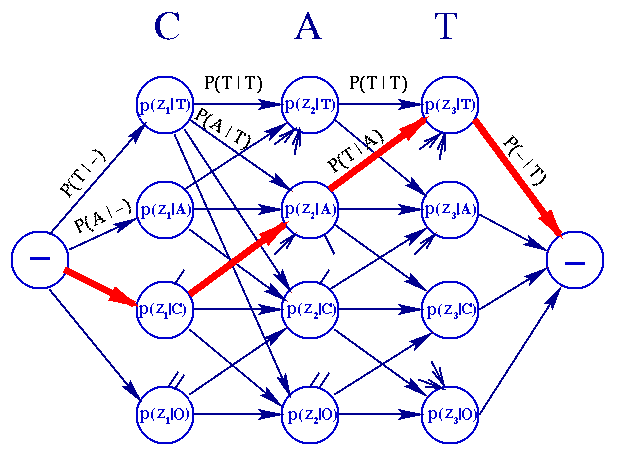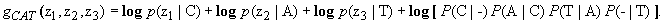The Text Recognition Problem

The goal of the text recognition problem is, as its name indicates, to achieve efficiently the recognition of characters from a text source; example of text sources are books, magazines, letters, etc. Nowadays, Optical Character Readers (OCR) are machines widely used to solve this problem, and their use is increasing even more. However, it has been noted that the use of contextual information might improve the reliability of OCR machines. Our own ability to understand misspelled words or ambiguities in English supports this conjecture. Examples of this fact are shown in Fig. 6. Almost in an unsconscious way, we use our vocabulary, the context of the sentcnce and our knowledge of spelling patterns to help us to find a word that fits the sentence and is similar to the one we see. Right now you probably noted that the word "sentence" was misspelled above by putting the letter "c" instead of "e". Maybe you did not notice it at all, or you did, but your brain worked so quickly, first by noticing that this word does not exist in the English vocabulary and second by matching it with the right word, that you did not get lost in the context.Fig. 6 Examples of the use the context in text recognition.

An OCR machine scans individual characters, isolates salient features, and then some decision is made as to what letter or other character lies below the reader. When the characters actually occur as part of natural-language text, it has long been recognized that contextual information can be used to assist the reader in resolving ambiguities. We mentioned six classes of word features that extract contextual information in the introduction section, and by intuition we can say that it is easier for a computer to use graphological, statistical and syntactic information than the other types. Efforts have been focused mainly on using statistical an syntactic information. The approaches that have been taken can be categorized as follows:Dictionary look-up methodsMarkov and Probability distribution approximation techniquesHybrid methods

All these methods are classified under the general statistical framework of compound decision theory, which was stated in the previous section. We proceed to describe briefly the first approach, and focus more on the second one, since it is in this category where the Viterbi algorithm is placed. The last one is worthly to be considered too, so the general idea will be just mentioned.

Dictionary look-up methods

A dictionary containing all forms of words which are to be considered legal words of the language can be used to validate the OCR results by matching them with the words in the dictionary. In the simplest form, the corresponding OCR words are only considered valid when they exist in the dictionary (exact matching). Otherwise, if the input word is not in the dictionary, correction techniques applying a similarity measure are needed for the improvement of recognition results (best matching).

This approach corrects errors very well but its main disadvantage is the amount of storage and computation required to store and search a dictionary. Access time is a crucial point when the dictionary size is large, for example, containing more than a hundred thousand words.

If you are interested in see in more detail how these methods work, you can check these sites out.

Markov and probability distribution approximation methods

This approach models a natural language like English as though it were a finite-state discrete-time n-th order Markov process and hence no dictionary is needed. Instead of using full knowledge about the allowed words of the vocabulary, they approximate this knowledge by enumerating the possible character transitions and by integrating their probabilities. Therefore, statistical information about the language is used, such as the frequency of letter pairs (bigrams) or letter trios (trigrams). In general, we can suppose that the probability of occurrence of each letter depends on the n previous letters, and estimate these probabilities from the frequencies of (n+1)-letter combinations: (n+1)-grams. Thus, for example, if the n=1 only the bigram transition probabilities are needed. The motivation for this method is the redundancy of a language. Previous analysis  has shown that only 70% of all bigrams occur in English textbooks. The percentage of trigrams occurring is even smaller.

An elegant Markov technique in which a decision is made on one word at a time is through the use of the Viterbi algorithm. The Viterbi algorithm provides an efficient way of finding the most likely sequence of characters in a maximum a posteriori (MAP) probability sense. To illustrate how the Viterbi algorithm works, we give a short example. We assume, a 4-letter alphabet, A={T,A,C,O}, and the observed string given by the OCR machine is Z= -CAT-, where "-" denotes blank or space. The features vectors z1, z2, and z3 are obtained when the feature extractor looks at C,A,T. The information available and relevant that the Viterbi algorithm traverses to make a decision on the word, is expressed in terms of a directed graph or trellis as in Fig. 7. All nodes (except the blank nodes "-") and edges have probabilities associated with them.Fig. 7 Trellis illustrating the example. Click on the image.

The edge probabilities (Markov transition probabilities between letters) remain fixed no matter what sequence of letters is presented to the machine, that is, they represent static information. The node probabilities (likelihood of the feature vectors obtained from the characters), on the other hand, are a function on the actual characters presented to the machine, that is, they represent dynamic information. We see from Fig. 7 that any path from the start node to the end node (the "-" nodes) represents a sequence of letters but not necessarily a valid word. Consider the red path in Fig. 7, which represent the letter sequence CAT. The aim is to find the letter sequence which maximizes the product of the probabilities of its corresponding path or, if viewed from the point of view of assigned lengths as stated in the previous section: find the shortest path that minimizes the total lenght of all paths. This can be done by processing the vertical node layers step by step from left to right. Hence, if we add the logarithms of all the edge and node probabilities encountered on that path we obtainWith such a model, English letters are viewed as the outputs of an M-state Markov process, where M is the number of distinguishable characters, such as 26 letters. At each stage in the sequence (word), 26 most likely sequences are computed, the most likely sequence ending in A, the most likely sequence ending in B, etc. At the final stage the most likely sequence is chosen.

The Viterbi algorithm can also be used with a confusion matrix, rather than the likelihood probabilities. A confusion matrix reports the number of each kind of error produced by an OCR on a training sample of text. Modifications of the Viterbi algorithm have been proposed for the sake of computational efficiency such as using logarithms of probabilities and their summation rather than their product , or by defining a variable number of alternatives by which the observed characters can be substituted . In general, it has been shown that the statistical approach is better for error correction.

Hybrid methods

Hybrid methods aim to combine the advantages of dictionary and statistical techniques. Markov models in the form of the Viterbi algorithm or its variants are used to generate likely word alternatives to the input. At each step, the corresponding substrings are then validated against the dictionary to check whether they constitute a valid word prefixed or not. Hence, incorrect word alternatives are avoided. If the input word, however, is not found in the dictionary, the Markov model can be applied to obtain the most likely word candidates.